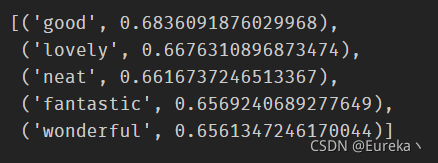﻿ 关于Word2Vec可视化展示_python_脚本之家
python# 关于Word2Vec可视化展示

## Word2Vec简介

Word2Vec是一个词嵌入过程。这个概念相对简单：通过一个句子一个句子地在语料库中循环去拟合一个模型，根据预先定义的窗口中的相邻单词预测当前单词。

Word2Vec中有两种建模方法：skip-gram和continuous bag of words，这两种方法都有各自的优点和对某些超参数的敏感性。

## 简单可视化

```import gensim

%matplotlib inline
from gensim.models import Word2Vec
from sklearn.decomposition import PCA
from matplotlib import pyplot

# 训练的语料
sentences = [['this', 'is', 'the', 'an', 'apple', 'for', 'you'],
['this', 'is', 'the', 'an', 'orange', 'for', 'you'],
['this', 'is', 'the', 'an', 'banana', 'for', 'you'],
['apple','is','delicious'],
['orange','is','delicious'],
['apple','tests','delicious'],
['orange','tests','delicious']]

# 利用语料训练模型
model = Word2Vec(sentences,window=5, min_count=1)

# 基于2d PCA拟合数据
# X = model[model.wv.vocab]
X = model.wv[model.wv.key_to_index]
pca = PCA(n_components=2)
result = pca.fit_transform(X)

# 可视化展示
pyplot.scatter(result[:, 0], result[:, 1])
words = list(model.wv.key_to_index)
for i, word in enumerate(words):
pyplot.annotate(word, xy=(result[i, 0], result[i, 1]))
pyplot.show()```## 实战演练

```from gensim.models.keyedvectors import KeyedVectors

binary = True, limit = 1000000)```

1、可以实际查看任意单词的向量表示：

`word_vectors['dog']`但很难解释这个向量的每一维代表什么意思。

2、可以使用most_similar函数找到意思相近的单词，topn参数定义要列出的单词数：

`word_vectors.most_similar(positive = ['nice'], topn = 5)`3、如果我们想合并father和woman这两个单词的向量，并减去man这个单词的向量，可以得到：

```word_vectors.most_similar(
positive = ['father', 'woman'], negative = ['man'], topn = 1)```4、可视化：

```%matplotlib inline
import pandas as pd
import matplotlib.pyplot as plt
import seaborn as sns
from sklearn.decomposition import PCA
```from jupyterthemes import jtplot
jtplot.style(theme='onedork') #选择一个绘图主题```
```def plot_2d_representation_of_words(
word_list,
word_vectors,
flip_x_axis = False,
flip_y_axis = False,
label_x_axis = "x",
label_y_axis = "y",
label_label = "fruit"):

pca = PCA(n_components = 2)

word_plus_coordinates=[]

for word in word_list:
current_row = []
current_row.append(word)
current_row.extend(word_vectors[word])
word_plus_coordinates.append(current_row)

word_plus_coordinates = pd.DataFrame(word_plus_coordinates)

coordinates_2d = pca.fit_transform(
word_plus_coordinates.iloc[:,1:300])
coordinates_2d = pd.DataFrame(
coordinates_2d, columns=[label_x_axis, label_y_axis])
coordinates_2d[label_label] = word_plus_coordinates.iloc[:,0]
if flip_x_axis:
coordinates_2d[label_x_axis] = \
coordinates_2d[label_x_axis] * (-1)
if flip_y_axis:
coordinates_2d[label_y_axis] = \
coordinates_2d[label_y_axis] * (-1)

plt.figure(figsize = (15,10))
p1=sns.scatterplot(
data=coordinates_2d, x=label_x_axis, y=label_y_axis)

x = coordinates_2d[label_x_axis]
y = coordinates_2d[label_y_axis]
label = coordinates_2d[label_label]

texts = [plt.text(x[i], y[i], label[i]) for i in range(len(x))]
```fruits = ['apple','orange','banana','lemon','car','tram','boat','bicycle',
'cherry','mango','grape','durian','watermelon','train','motorbike','ship',
'peach','pear','pomegranate','strawberry','bike','bus','truck','subway','airplane']```
```plot_2d_representation_of_words(
word_list = fruits,
word_vectors = word_vectors,
flip_y_axis = True)```· 计算相似度

• 寻找相似词
• 信息检索

· 作为SVM/LSTM等模型的输入

• 中文分词
• 命名体识别

· 句子表示

• 情感分析

· 文档表示

• 文档主题判别